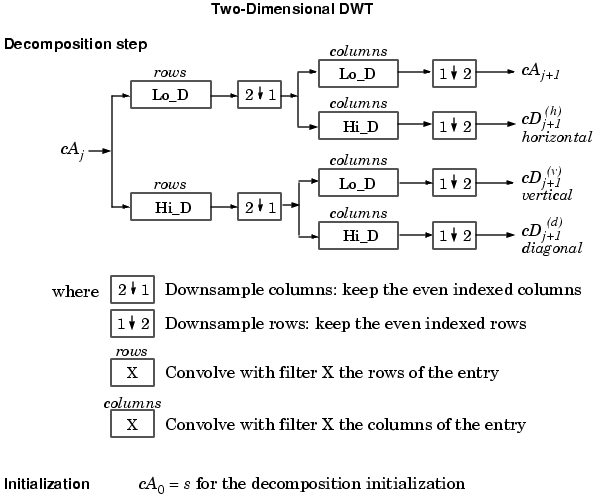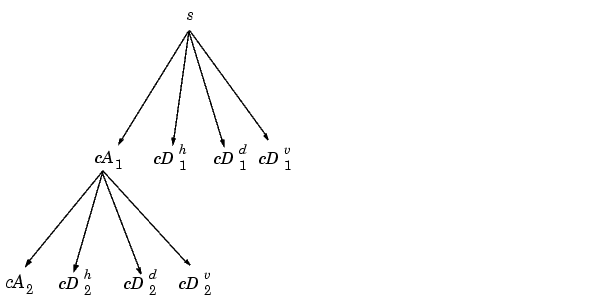Wavelet Toolboxwavedec2

Multilevel 2-D wavelet decomposition

Syntax

• ```[C,S] = wavedec2(X,N,'`wname`')
[C,S] = wavedec2(X,N,Lo_D,Hi_D)
```

Description

`wavedec2` is a two-dimensional wavelet analysis function.

`[C,S] = wavedec2(X,N,`'`wname`'`)` returns the wavelet decomposition of the matrix `X` at level `N`, using the wavelet named in string '`wname`' (see `wfilters` for more information).

Outputs are the decomposition vector `C` and the corresponding bookkeeping matrix `S`.

`N` must be a strictly positive integer (see `wmaxlev` for more information).

Instead of giving the wavelet name, you can give the filters.

For `[C,S] = wavedec2(X,N,`Lo_D,Hi_D`)`, Lo_D is the decomposition low-pass filter and Hi_D is the decomposition high-pass filter.

Vector `C` is organized as

`C = [ A(N) | H(N) | V(N) | D(N) | ... `

`H(N-1) | V(N-1) | D(N-1) | ... | H(1) | V(1) | D(1) ].`

where `A`, `H`, `V`, `D`, are row vectors such that

`A` = approximation coefficients

`H` = horizontal detail coefficients

`V` = vertical detail coefficients

`D` = diagonal detail coefficients

Each vector is the vector column-wise storage of a matrix.

Matrix `S` is such that

`S(1,:) `= size of approximation coefficients(`N`)

`S(i,:) `= size of detail coefficients(`N-i+2`) for `i `= 2, ...`N+1` and
`S(N+2,:) = size(X)`Examples

• ```% The current extension mode is zero-padding (see `dwtmode`).

% X contains the loaded image.

% Perform decomposition at level 2
% of X using db1.
[c,s] = wavedec2(X,2,'db1');

% Decomposition structure organization.
sizex = size(X)

sizex =
256  256
sizec = size(c)

sizec =
1  65536
val_s = s

val_s =
64  64
64  64
128  128
256  256
```

Algorithm

For images, an algorithm similar to the one-dimensional case is possible for two-dimensional wavelets and scaling functions obtained from one-dimensional ones by tensor product.

This kind of two-dimensional DWT leads to a decomposition of approximation coefficients at level j in four components: the approximation at level j+1, and the details in three orientations (horizontal, vertical, and diagonal).

The following chart describes the basic decomposition step for images:So, for J=2, the two-dimensional wavelet tree has the form`dwt`, `waveinfo`, `waverec2`, `wfilters`, `wmaxlev`wavedec wavedemo